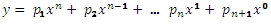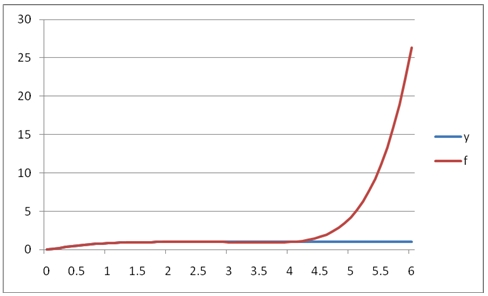# SQL Server polynomial value

POLYVAL

Updated: 30 April 2011

Use POLYVAL for calculating a new y-value given a new x-value using the coefficients of a polynomial p(x) of degree n that fits the x- and y-values supplied to the function. The y-value is calculated using n+1 polynomial coefficients in descending powers:SyntaxArguments
@known_x
the x-values to be used in the calculation. @known_x must be of the type float or of a type that implicitly converts to float.
@known_y
the y-values to be used in the calculation. @known_y must be of the type float or of a type that implicitly converts to float.
@degree
an integer specifying the degree of the polynomial.
@new_x
the new x-value for which you want POLYVAL to calculate the y-value.
Return Types
float
Remarks
·         The x- and y-values are passed to the function as pairs.
·         If x is NULL or y is NULL, the pair is not used in the calculation.
·         Use the POLYFIT or POLYFIT_q functions to get the coefficients.
·         @degree must remain invariant for the GROUP.
·         @new_x must remain invariant for the GROUP.
·         Available in XLeratorDB / math 2008 only
Examples
In this example, we will use the westclintech SERIESFLOAT function to generate a series of x-values equally spaced in the interval [0, 2.5] and then evaluate the error function, ERF, at those points. We will specify an approximating polynomial of 6 degrees. We will then generate values at the midpoint for each interval using the approximating polynomial
SET NOCOUNT ON

SELECT SeriesValue as x
,westclintech.wct.ERF(SeriesValue) as y
INTO #erf
FROM wct.SeriesFloat(0,2.5,0.1,NULL,NULL)

SELECT a.seriesvalue as x
,wct.POLYVAL(e.x, e.y, 6, seriesvalue) as POLYVAL
FROM wct.SERIESFLOAT(0.05,2.5,0.1,NULL,NULL) a
,#erf E
GROUP BY seriesvalue

DROP TABLE #erf
This produces the following result.
x                POLYVAL
---------------------- ----------------------
0.05     0.0560409087420763
0.15      0.167414870029564
0.25      0.276183548384518
0.35      0.379669434495585
0.45      0.475932241043269
0.55      0.563669129052707
0.65      0.642120996194105
0.75      0.710984827030842
0.85      0.770332105215247
0.95       0.82053328763204
1.05      0.862188340489436
1.15      0.896063337357925
1.25      0.923033119156717
1.35      0.944030016087848
1.45      0.959998631517963
1.55      0.971856687807761
1.65      0.980461934089109
1.75      0.986585115989822
1.85      0.990889007306113
1.95      0.993913503622709
2.05      0.996066777880637
2.15      0.997622497892672
2.25      0.998723105806456
2.35      0.999389159515293
2.45      0.999534736016589
Since we know that the y-values are actually erf(x), in this example we will compare the values calculated using the approximating polynomial and erf(x).
SET NOCOUNT ON

SELECT SeriesValue as x
,westclintech.wct.ERF(SeriesValue) as y
INTO #erf
FROM wct.SeriesFloat(0,2.5,0.1,NULL,NULL)

SELECT x
,POLYVAL
,[ERF(x)]
,POLYVAL - [ERF(x)] as [DIFFERENCE]
FROM (
SELECT a.seriesvalue as x
,wct.POLYVAL(e.x, e.y, 6, a.seriesvalue) as POLYVAL
,westclintech.wct.ERF(a.SeriesValue) as [ERF(x)]
FROM wct.SERIESFLOAT(0.05,2.5,0.1,NULL,NULL) a
,#erf E
GROUP BY seriesvalue
) m

DROP TABLE #erf
This produces the following result.
x                POLYVAL                 ERF(x)             DIFFERENCE
------- ---------------------- ---------------------- ----------------------
0.05    0.0560409087420763     0.0563719777970165   -0.00033106905494016
0.15      0.167414870029564      0.167995971427363 -0.000581101397799128
0.25      0.276183548384518      0.276326390168236 -0.000142841783717984
0.35      0.379669434495585      0.379382053562309    0.00028738093327535
0.45      0.475932241043269      0.475481719786923   0.000450521256346315
0.55      0.563669129052707      0.563323366325108   0.000345762727599452
0.65      0.642120996194105      0.642029327355671   9.16688384339226E-05
0.75      0.710984827030842      0.711155633653513 -0.000170806622671549
0.85      0.770332105215247      0.770668057608351 -0.000335952393103467
0.95       0.82053328763204      0.820890807273276 -0.000357519641236315
1.05      0.862188340489436      0.862436106090095 -0.000247765600658978
1.15      0.896063337357925      0.896123842936913 -6.05055789882902E-05
1.25      0.923033119156717      0.922900128256456   0.000132990900260643
1.35      0.944030016087848      0.943762196122722   0.000267819965126148
1.45      0.959998631517963      0.959695025637457   0.000303605880506042
1.55      0.971856687807761       0.97162273326201   0.000233954545751147
1.65      0.980461934089109      0.980375585023358   8.63490657508903E-05
1.75      0.986585115989822       0.98667167121918 -8.65552293580762E-05
1.85      0.990889007306113      0.991111030056084 -0.000222022749971074
1.95      0.993913503622709      0.994179333592187 -0.000265829969478215
2.05      0.996066777880637      0.996258096044454 -0.000191318163817344
2.15      0.997622497892672      0.997638607037322 -1.61091446494455E-05
2.25      0.998723105806456      0.998537283413317   0.000185822393138357
2.35      0.999389159515293      0.999110732967865   0.000278426547427824
2.45      0.999534736016589      0.999469419887746   6.53161288429738E-05

It looks like the approximating polynomial is accurate to about 3 or 4 decimal places. In fact if we graphed the results, they would look like this:We can see that in this range, [0, 2.5] the fit between the y-values and the f-values (ERF(x) in this case) is quite good.
Since POLYVAL creates an approximating polynomial, it is not bound by minima and maxima of x-y pairs. In this example, we will graph the results this SQL, which will using the approximating polynomial from the [0, 2.5] interval on the interval [0, 6]
SET NOCOUNT ON

SELECT SeriesValue as x
,westclintech.wct.ERF(SeriesValue) as y
INTO #erf
FROM wct.SeriesFloat(0,2.5,0.1,NULL,NULL)

SELECT a.seriesvalue as x
,wct.POLYVAL(e.x, e.y, 6, seriesvalue) as POLYVAL
FROM wct.SERIESFLOAT(0.0,6.0,0.1,NULL,NULL) a
,#erf E
GROUP BY seriesvalue

DROP TABLE #erf
This produces the following graph.As you can see, the polynomial approximation quickly diverges from the function in the region above 2.5.
Let’s look at how POLYVAL works with dates. In this example we will look at some interest rate values over a time-horizon and calculate the 3rd degree polynomial approximation. The x-values are dates and the y-values are the decimal values of the interest rate (.01 = 1%).
SET NOCOUNT ON

SELECT cast(x as datetime) as x,y
INTO #a
FROM (VALUES
('2012-Apr-30',0.0028),
('2013-Apr-30',0.0056),
('2014-Apr-30',0.0085),
('2016-Apr-30',0.0164),
('2018-Apr-30',0.0235),
('2021-Apr-30',0.0299),
('2031-Apr-30',0.0382),
('2041-Apr-30',0.0406)
) m(x, y)

SELECT wct.POLYVAL(cast(x as float), y, 3, cast(Cast('2012-Oct-31' as datetime) as float)) as POLYVAL
from #a

DROP TABLE #a

This produces the following result.
POLYVAL
----------------------
0.00378351662681808

In this example we will use POLYVAL, the SERIESINT function and the EOMONTH function from XLeratorDB/financial to calculate the rates for each year from 1 through 30 out to 4 decimal places.
SET NOCOUNT ON

SELECT cast(x as datetime) as x,y
INTO #a
FROM (VALUES
('2012-Apr-30',0.0028),
('2013-Apr-30',0.0056),
('2014-Apr-30',0.0085),
('2016-Apr-30',0.0164),
('2018-Apr-30',0.0235),
('2021-Apr-30',0.0299),
('2031-Apr-30',0.0382),
('2041-Apr-30',0.0406)
) m(x, y)

SELECT convert(varchar,westclintech.wct.EOMONTH('04/30/2011', SeriesValue), 106) as [MATURITY DATE]
,ROUND(wct.POLYVAL(cast(x as float), y, 3, cast(westclintech.wct.EOMONTH('04/30/2011', SeriesValue) as float)), 4) as [INTEREST RATE]
from #a, wct.SeriesInt(12,360,12,NULL,NULL)
GROUP BY westclintech.wct.EOMONTH('04/30/2011', SeriesValue)

DROP TABLE #a
This produces the following result.
MATURITY DATE                           INTEREST RATE
------------------------------ ----------------------
30 Apr 2012                                    0.0015
30 Apr 2013                                    0.0059
30 Apr 2014                                    0.0099
30 Apr 2015                                    0.0136
30 Apr 2016                                     0.017
30 Apr 2017                                      0.02
30 Apr 2018                                    0.0228
30 Apr 2019                                    0.0252
30 Apr 2020                                    0.0274
30 Apr 2021                                    0.0293
30 Apr 2022                                     0.031
30 Apr 2023                                    0.0325
30 Apr 2024                                    0.0338
30 Apr 2025                                    0.0349
30 Apr 2026                                    0.0358
30 Apr 2027                                    0.0366
30 Apr 2028                                    0.0373
30 Apr 2029                                    0.0378
30 Apr 2030                                    0.0382
30 Apr 2031                                    0.0385
30 Apr 2032                                    0.0388
30 Apr 2033                                     0.039
30 Apr 2034                                    0.0391
30 Apr 2035                                    0.0393
30 Apr 2036                                    0.0394
30 Apr 2037                                    0.0395
30 Apr 2038                                    0.0397
30 Apr 2039                                    0.0399
30 Apr 2040                                    0.0402
30 Apr 2041                                    0.0405

### SupportCopyright 2008-2021 Westclintech LLC         Privacy Policy        Terms of Service